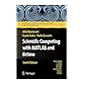Normal view

Scientific Computing with MATLAB and Octave [electronic resource] : Second Edition / by Alfio Quarteroni, Fausto Saleri.

Material type:TextPublisher: Berlin, Heidelberg : Springer Berlin Heidelberg, 2006Description: XVI, 318 p. 108 illus., 97 illus. in color. online resourceContent type: text Media type: computer Carrier type: online resourceISBN: 9783540326137Additional physical formats: Printed edition:: No titleDDC classification: 004.0151 LOC classification: QA75.5-76.95Online resources: Click here to access online
Contents:
What can’t be ignored -- Nonlinear equations -- Approximation of functions and data -- Numerical differentiation and integration -- Linear systems -- Eigenvalues and eigenvectors -- Ordinary differential equations -- Numerical methods for (initial-)boundary-value problems -- Solutions of the exercises.
Summary: This textbook is an introduction to Scientific Computing, in which several numerical methods for the computer solution of certain classes of mathematical problems are illustrated. The authors show how to compute the zeros or the integrals of continuous functions, solve linear systems, approximate functions by polynomials and construct accurate approximations for the solution of ordinary and partial differential equations. To make the presentation concrete and appealing, the programming environments Matlab and Octave, which is freely distributed, are adopted as faithful companions. The book contains the solutions to several problems posed in exercises and examples, often originating from specific applications. A specific section is devoted to subjects which were not addressed in the book and contains the bibliographical references for a more comprehensive treatment of the material. The second edition features many new problems and examples, as well as more numerical methods for linear and nonlinear systems and ordinary and partial differential equations.
Tags from this library: No tags from this library for this title.Average rating: 0.0 (0 votes)
Item type Current location Collection Call number Status Date due Barcode Item holdseBook e-Library

Electronic Book@IST

EBook Available
Total holds: 0

What can’t be ignored -- Nonlinear equations -- Approximation of functions and data -- Numerical differentiation and integration -- Linear systems -- Eigenvalues and eigenvectors -- Ordinary differential equations -- Numerical methods for (initial-)boundary-value problems -- Solutions of the exercises.

This textbook is an introduction to Scientific Computing, in which several numerical methods for the computer solution of certain classes of mathematical problems are illustrated. The authors show how to compute the zeros or the integrals of continuous functions, solve linear systems, approximate functions by polynomials and construct accurate approximations for the solution of ordinary and partial differential equations. To make the presentation concrete and appealing, the programming environments Matlab and Octave, which is freely distributed, are adopted as faithful companions. The book contains the solutions to several problems posed in exercises and examples, often originating from specific applications. A specific section is devoted to subjects which were not addressed in the book and contains the bibliographical references for a more comprehensive treatment of the material. The second edition features many new problems and examples, as well as more numerical methods for linear and nonlinear systems and ordinary and partial differential equations.

There are no comments for this item.Scientific computing with MATLAB and Octave by Quarteroni, Alfio